International
Tables for
Crystallography
Volume A1
Symmetry relations between space groups
Edited by Hans Wondratschek and Ulrich Müller

International Tables for Crystallography (2006). Vol. A1, ch. 1.3, pp. 25-26   | 1 | 2 |

## Section 1.3.4. Relations between the positions in group–subgroup relations

Ulrich Müllera*

aFachbereich Chemie, Philipps-Universität, D-35032 Marburg, Germany
Correspondence e-mail: mueller@chemie.uni-marburg.de

### 1.3.4. Relations between the positions in group–subgroup relations

| top | pdf |

The following statements are universally valid:

 (1) Between the points of an orbit and the corresponding points in a subgroup there exists a one-to-one relation; both sets of points have the same magnitude. (2) Between the Wyckoff positions of a space group and those of its subgroups there exist unique relations. These may involve different Wyckoff labels for different relative positions of the origins. (3) With the symmetry reduction from a group to a subgroup, an orbit either splits into different orbits, or its site symmetry is reduced, or both happen. In addition, coordinates fixed or coupled by symmetry may become independent.

Letbe a space group anda subgroup of. Let the site-symmetry groups of a point X under the space groupsandbe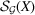and, respectively. The reduction factor of the site symmetries is then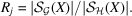When the space-group symmetry is reduced fromtoand the orbit of the point X splits into n orbits, the following relation holds (Wondratschek, 2005):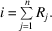is the index ofin(cf. Section 1.2.4.2).

#### Example 1.3.4.1.

The orbit of the Wyckoff position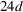of space group, No. 225, has the site symmetrywith the order. Upon symmetry reduction to the space group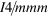, No. 139, this orbit splits into the two orbits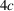andofwith the site symmetriesand, respectively.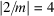. The reduction factors of the site symmetries are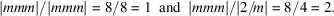They add up to, which is the index ofin.

The multiplicities commonly used together with the Wyckoff labels depend on the size of the chosen unit cell. As a consequence, a change of the size of the unit cell also changes the multiplicities. For example, the multiplicities of the Wyckoff positions listed in Volume A are larger by a factor of three for rhombohedral space groups when the unit cell is referred not to rhombohedral, but to hexagonal axes.

The multiplicity of a Wyckoff position shows up in the sum of the multiplicities of the corresponding positions of the subgroup. If the unit cell selected to describe the subgroup does not change in size, then the sum of the multiplicities of the positions of the subgroup must be equal to the multiplicity of the position of the starting group. For example, from a position with a multiplicity of 6, a position with multiplicity of 6 can result, or it can split into two positions of multiplicity of 3, or into two with multiplicities of 2 and 4, or into three with multiplicity of 2 etc. If the unit cell of the subgroup is enlarged or reduced by a factor f, then the sum of the multiplicities must also be multiplied or divided by this factor f.

Relations between the Wyckoff positions of space groups and the Wyckoff positions of their maximal subgroups were listed by Lawrenson (1972). However, his tables are not complete, and they were never published. In addition, they lack information about the transformations of axes and coordinates when these differ in the subgroup.

More recently, a computer program to calculate these relations has been developed (Kroumova et al., 1998). To be used, the program requires knowledge of the subgroups (maximal or non-maximal) and of the necessary axes transformations and origin shifts. The Wyckoff position(s) to be considered can be marked or specific coordinates of a position must be given. The output is a listing of the Wyckoff position(s) of the specified subgroup and optionally all corresponding site coordinates. Depending on the relations and positions considered, the listings of coordinates can be rather long. The program has not been designed to give a fast overview of the relations. If one is looking for those subgroups that will exhibit a splitting of a certain position, all subgroups have to be tried one by one. For these reasons, the program cannot substitute the present tables; for practical work, the program and the tables listed in Part 3complement each other.

The tables in Part 3are a complete compilation for all space groups and all of their maximal subgroups. For all Wyckoff positions of a space group, all relations to the Wyckoff positions of its subgroups are listed. This also applies to the infinite number of maximal isomorphic subgroups; for these, a parameterized form has been developed that allows the listing of all maximal subgroups and all of their resulting Wyckoff positions completely for every allowed index of symmetry reduction.

### References

Kroumova, E., Perez-Mato, J. M. & Aroyo, M. I. (1998). WYCKSPLIT. Computer program for the determination of the relations of Wyckoff positions for a group–subgroup pair. J. Appl. Cryst. 31, 646. Accessible at: http://www.cryst.ehu.es/cryst/wpsplit.html .Google Scholar
Lawrenson, J. E. (1972). Theoretical studies in crystallography. Dissertation, University of Kent at Canterbury.Google Scholar
Wondratschek, H. (2005). Special topics on space groups. International tables for crystallography, Vol. A, Space-group symmetry, edited by Th. Hahn, Part 8. Heidelberg: Springer.Google Scholar